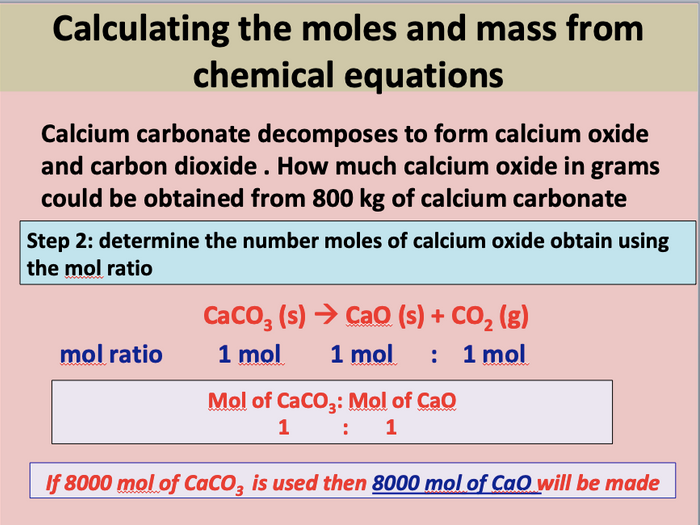A complete lesson including starter activity, mini AfL work tasks with answers, main work tasks with answers for a KS5 lesson on reacting masses (moles and chemical equations)

By the end of the lesson students should be able to:

Know how to balance symbol equations
Calculate the moles of reactants or products based on chemical equations and mole ratios
Calculate the masses of reactants used or products formed based on chemical equations and mole ratios

Students will be able to take rich notes on reacting masses, building on their KS4 knowledge on this topic

The teacher will be able to quickly assess students’ understanding of the how to balanced equations and calculate reacting masses from chemical equations by carrying our mini AfL tasks either on mini white boards or in students’ books

The lesson ends with a main work task for students to complete. Students will be able to self or peer assess their answers to this task using the detailed answers provided

\$9.07
Save for later

### Info

Created: Nov 25, 2018

Updated: Oct 13, 2019

pptx, 440 KB

#### Worksheet

pptx, 52 KB

rules-for-balancing-equations-

pptx, 65 KB

Starter-quiz-

Report a problem

### Tes Paid Licence

How can I re-use this?